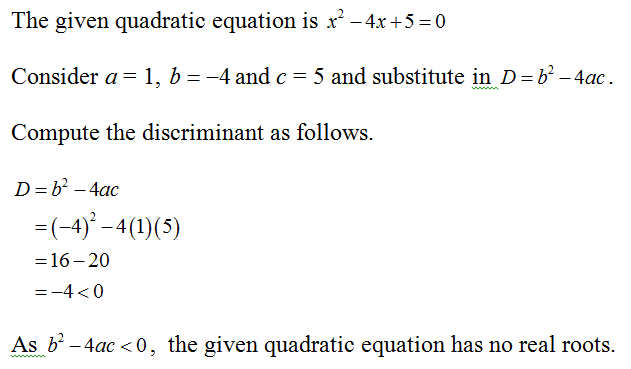# Use the discriminant (D=b2-4ac) to determine the type of solutions of each of the following quadratic equations and then determine the solutions and also determine the discriminant. 5. x2-4x+5=0

Question
3 views

Use the discriminant (D=b2-4ac) to determine the type of solutions of each of the following quadratic equations and then determine the solutions and also determine the discriminant.
5. x2-4x+5=0

check_circle

Step 1...

### Want to see the full answer?

See Solution

#### Want to see this answer and more?

Solutions are written by subject experts who are available 24/7. Questions are typically answered within 1 hour.*

See Solution
*Response times may vary by subject and question.
Tagged in